2017-11-24 22:42:45 xxxqcbQ 阅读数 245
• ###### Java经典算法讲解

在面试中，算法题目是必须的，通过算法能够看出一个程序员的编程思维，考察对复杂问题的设计与分析能力，对问题的严谨性都能够体现出来。一个算法的好坏，直接影响一个方法调用的性能，进而影响软件的整体性能。算法是学习所有编程语言的基础，在Java的学习过程中首先也会选择以算法起步，本次课程重点讲解Java开发中常用的基本算法。

29971 人正在学习 去看看 张中强

一直想记录自己图像处理算法修炼心路历程，今天终于鼓足勇气写下第一篇，万事开头难。

算法写了快4个月了，目前写的比较多的是基础算法，比如通用的圆、直线检测算法，还真不能小瞧这类算法，想在工程上做到又快又准，真的不是一件容易的事情。这里应该算是有bug的，又快又好应当有个明确的量化指标。

对于圆检测的基本逻辑是：Sobel/Canny算子处理→拿出边界→边界筛选→最小二乘拟合(对噪声很敏感)。

2016-06-06 17:34:31 xueyedie1234 阅读数 23290
• ###### Java经典算法讲解

在面试中，算法题目是必须的，通过算法能够看出一个程序员的编程思维，考察对复杂问题的设计与分析能力，对问题的严谨性都能够体现出来。一个算法的好坏，直接影响一个方法调用的性能，进而影响软件的整体性能。算法是学习所有编程语言的基础，在Java的学习过程中首先也会选择以算法起步，本次课程重点讲解Java开发中常用的基本算法。

29971 人正在学习 去看看 张中强

### 文章目录

# 一、 什么是卷积？       在图像处理中，卷积操作指的是使用一个卷积核对图像中的每个像素进行一系列操作。       卷积核（算子）是用来做图像处理时的矩阵,图像处理时也称为掩膜，是与原图像做运算的参数。卷积核通常是一个四方形的网格结构（例如3*3的矩阵或像素区域），该区域上每个方格都有一个权重值。       使用卷积进行计算时，需要将卷积核的中心放置在要计算的像素上，一次计算核中每个元素和其覆盖的图像像素值的乘积并求和，得到的结构就是该位置的新像素值。       以下两个算子中演示了具体的卷积计算过程。
# 二、 相关算子 定义：![这里写图片描述](http://img.blog.csdn.net/20160603125126517) 即![这里写图片描述](http://img.blog.csdn.net/20160603125155767)，其中ｈ称为相关核(Kernel). 　　　　　　　　 步骤：
• 1）滑动核，使其中心位于输入图像g的（i，j）像素上
• 2）利用上式求和，得到输出图像的（i，j）像素值
• 3）充分上面操纵，直到求出输出图像的所有像素值

【例】
原始像素矩阵为：
\begin{bmatrix}
17 & 24 & 1 & 8 & 15 \
23 & 5 & 7 & 14 & 16 \
4 & 6 & 13 & 20 & 22 \
10 & 12 & 19 & 21 & 3\
11 & 18 & 25 & 2 & 9
\end{bmatrix}

\begin{bmatrix}
8 & 1 & 6 \
3 & 5 & 7 \
4 & 9 & 2
\end{bmatrix}# 三、 卷积算子• 1）将核围绕中心旋转180度
• 2）滑动核，使其中心位于输入图像g的（i，j）像素上
• 3）利用上式求和，得到输出图像的（i，j）像素值
• 4）充分上面操纵，直到求出输出图像的所有像素值
例：计算输出图像的（2，4）元素=12+89+154+77+145+163+136+201+22*8=575
如图所示：# 四、 边缘效应       当对图像边缘的进行滤波时，核的一部分会位于图像边缘外面。 ![这里写图片描述](http://img.blog.csdn.net/20160603143253118) 常用的策略包括： - 1）使用常数填充：imfilter默认用0填充，这会造成处理后的图像边缘是黑色的。 - 2）复制边缘像素：I3 = imfilter(I,h,'replicate'); ![这里写图片描述](http://img.blog.csdn.net/20160603143353525)
# 五、 常用的卷积核及其用途
• 1）低通滤波器（常用于计算模糊后的效果）\begin{bmatrix}
1/9 & 1/9 & 1/9 \
1/9 & 1/9 & 1/9 \
1/9 & 1/9 & 1/9
\end{bmatrix}
\begin{bmatrix}
1/10 & 1/10 & 1/10 \
1/10 & 2/10 & 1/10 \
1/10 & 1/10 & 1/10
\end{bmatrix}
\begin{bmatrix}
1/16 & 2/16 & 1/16 \
2/16 & 4/16 & 2/16 \
1/16 & 2/16 & 1/16
\end{bmatrix}

• 2）高斯滤波器（常用于计算高斯模糊后的效果）
高斯模糊的卷积核也是一个正方形的滤波核，其中每个元素通过以下公式计算得出：
$G(x,y)=\frac{1}{2πσ^{2}}·e^{\frac{x^{2}+y^{2}}{2σ^{2}}}$
该公式中σ是标准方差（一般取值为1），x和y分别对应了当前位置到卷积核中心的整数距离。通过这个公式，就可以计算出高斯核中每个位置对应的值。为了保证滤波后的图像不会变暗，需要对高斯核中的权重进行归一化。

• 3）边缘检测（常用于计算图像边缘或者说梯度值）
\begin{bmatrix}
-1 & 0 & -1 \
0 & 4 & 0 \
-1 & 0 & -1
\end{bmatrix}

# 六、 一个例子——使用卷积实现模糊效果       我们将对下面这张图进行模糊处理： ![这里写图片描述](http://img.blog.csdn.net/20160606172529227)       以下为compute shader中关于卷积处理的代码：
[numthreads(32,32,1)]
void Dim_Main (uint3 id : SV_DispatchThreadID)
{

float sumR = 0;
float sumG = 0;
float sumB = 0;
float sumA = 0;
for (int i = -1; i <= 1; i++)
{
for (int j = -1; j <= 1; j++)
{
sumR += texBuffer[(id.x+i)*texWidth+(id.y+j)].r * convolutionTempBuffer[(i+1)*3+(j+1)];
sumG += texBuffer[(id.x+i)*texWidth+(id.y+j)].g * convolutionTempBuffer[(i+1)*3+(j+1)];
sumB += texBuffer[(id.x+i)*texWidth+(id.y+j)].b * convolutionTempBuffer[(i+1)*3+(j+1)];
sumA += texBuffer[(id.x+i)*texWidth+(id.y+j)].a * convolutionTempBuffer[(i+1)*3+(j+1)];
}
}

texBuffer[id.x*texWidth+id.y].r = sumR;
texBuffer[id.x*texWidth+id.y].g = sumG;
texBuffer[id.x*texWidth+id.y].b = sumB;
texBuffer[id.x*texWidth+id.y].a = sumA;

Result[id.xy] = float4(sumR, sumG, sumB, sumA);
}图中可以明显的看到左右两边有明显的黑色线条，原图中是没有这样的黑色的，产生这种效果的原因是本文中之前提到过的边缘效应。下面我将修改一部分代码去除边缘效应带来的影响，这里使用的是相邻像素的值方法。

[numthreads(32,32,1)]
void Dim_Main (uint3 id : SV_DispatchThreadID)
{

float sumR = 0;
float sumG = 0;
float sumB = 0;
float sumA = 0;
for (int i = -1; i <= 1; i++)
{
for (int j = -1; j <= 1; j++)
{
if((id.x+i)*texWidth+(id.y+j)>texWidth*texWidth-1)
{
sumR += texBuffer[(id.x+i)*texWidth+(id.y+j)-texWidth].r * convolutionTempBuffer[(i+1)*3+(j+1)];
sumG += texBuffer[(id.x+i)*texWidth+(id.y+j)-texWidth].g * convolutionTempBuffer[(i+1)*3+(j+1)];
sumB += texBuffer[(id.x+i)*texWidth+(id.y+j)-texWidth].b * convolutionTempBuffer[(i+1)*3+(j+1)];
sumA += texBuffer[(id.x+i)*texWidth+(id.y+j)-texWidth].a * convolutionTempBuffer[(i+1)*3+(j+1)];
}

sumR += texBuffer[(id.x+i)*texWidth+(id.y+j)].r * convolutionTempBuffer[(i+1)*3+(j+1)];
sumG += texBuffer[(id.x+i)*texWidth+(id.y+j)].g * convolutionTempBuffer[(i+1)*3+(j+1)];
sumB += texBuffer[(id.x+i)*texWidth+(id.y+j)].b * convolutionTempBuffer[(i+1)*3+(j+1)];
sumA += texBuffer[(id.x+i)*texWidth+(id.y+j)].a * convolutionTempBuffer[(i+1)*3+(j+1)];
}
}

texBuffer[id.x*texWidth+id.y].r = sumR;
texBuffer[id.x*texWidth+id.y].g = sumG;
texBuffer[id.x*texWidth+id.y].b = sumB;
texBuffer[id.x*texWidth+id.y].a = sumA;

Result[id.xy] = float4(sumR, sumG, sumB, sumA);
}可以看到，图中左边的黑色线条已经被滤除，右边也可以采用类似的方法来剔除。实际使用中，也可以根据情况使用纯色来做剔除，这样可以节省部分效率，如下图中我使用的是纯白色来剔除边缘效应。2019-03-29 14:18:20 tomy2426214836 阅读数 100685
• ###### Java经典算法讲解

在面试中，算法题目是必须的，通过算法能够看出一个程序员的编程思维，考察对复杂问题的设计与分析能力，对问题的严谨性都能够体现出来。一个算法的好坏，直接影响一个方法调用的性能，进而影响软件的整体性能。算法是学习所有编程语言的基础，在Java的学习过程中首先也会选择以算法起步，本次课程重点讲解Java开发中常用的基本算法。

29971 人正在学习 去看看 张中强

RGB颜色空间

RGB(red,green,blue)颜色空间最常用的用途就是显示器系统，彩色阴极射线管,彩色光栅图形的显示器 都使用R、G、B数值来驱动R、G、B 电子枪发射电子，并分别激发荧光屏上的R、G、B三种颜色的荧光粉发出不同亮度的光线，并通过相加混合产生各种颜色；扫描仪也是通过吸收原稿经反射或透射而发送来 的光线中的R、G、B成分，并用它来表示原稿的颜色。RGB色彩空间称为与设备相关的色彩空间，因为不同的扫描仪扫描同一幅图像，会得到不同色彩的图像数据；不同型号的显示器显示同一幅图像，也会有不同 的色彩显示结果。显示器和扫描仪使用的RGB空间与CIE 1931 RGB真实三原色表色系统空间是不同的，后者 是与设备无关的颜色空间。btw：Photoshop的色彩选取器(Color Picker)。可以显示HSB、RGB、LAB和CMYK 色彩空间的每一种颜色的色彩值。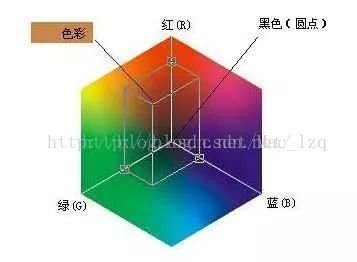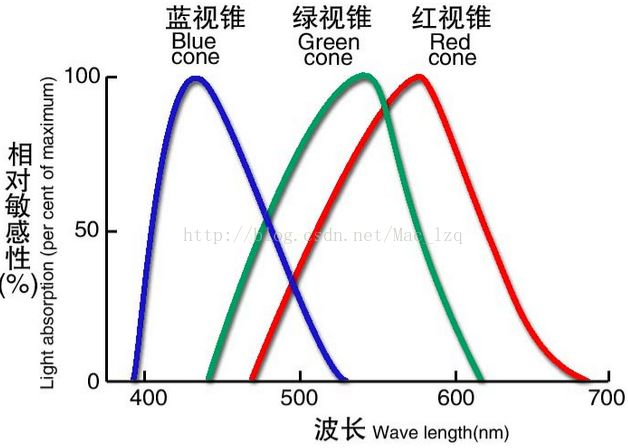RGB对应到显示器的三个刺激值，组成三维正交坐标系统，该系统中任何颜色都落入RGB彩色立方体内，在RGB颜色模型中，黑色在原点处，白色位于离原点最远的角上，灰度级沿着这两点的连线分布，每一个分量图像都是其原色图像。

RGB颜色空间最大的优点就是适合于显示系统，直观且容易理解。但是对彩色描述上的应用还有以下不足：

(1) RGB颜色空间利用三个颜色分量的线性组合来表示颜色，因此不同的色彩难以用精确的数值来表示，定量分析困难。

(2) 在RGB颜色系统中，三个颜色分量之间是高度相关的，即只要亮度改变，三个分量都会相应的改变，如果一个颜色的某一个分量发生了一定程度的改变，那么这颜色很可能也要发生改变。

(3) RGB颜色空间是一种均匀性较差的颜色空间，人眼对于三个颜色分量的敏感程度是不一样的，如果颜色的相似性直接用欧氏距离来度量，其结果与人眼视觉会有较大的偏差。

HSV颜色空间

HIS(Hue-Intensity-Saturation)颜色空间是图像处理中另外一个常用的颜色空间，它从人的视觉系统出发，用色调（Hue）、饱和度（Saturation或Chroma）和亮度（Intensity或Brightness）来描述颜色。HIS颜色空间可以用图3的圆锥空间模型来描述。其中，色调H由角度表示，其取值范围是 ，其中表示红色，表示黄色，表示绿色，表示蓝色，表示品红色。饱和度S是HIS彩色空间中轴线到彩色点的半径长度，彩色点离轴线的距离越近，表示颜色的白光越多。强度I用轴线方向上的高度表示，圆锥体的轴线描述了灰度级，强度最小值时为黑色，强度最大值时为白色。每个和轴线正交的切面上的点，其强度值都是相等的。

HSV(hue,saturation,value)颜色空间的模型对应于圆柱坐标系中的一个圆锥形子集，圆锥的顶面对应于V=1. 它包含RGB模型中的R=1，G=1，B=1 三个面，所代表的颜色较亮。色彩H由绕V轴的旋转角给定。红色对应于 角度0° ，绿色对应于角度120°，蓝色对应于角度240°。在HSV颜色模型中，每一种颜色和它的补色相差180° 。 饱和度S取值从0到1，所以圆锥顶面的半径为１。HSV颜色模型所代表的颜色域是CIE色度图的一个子集，这个 模型中饱和度为百分之百的颜色，其纯度一般小于百分之百。在圆锥的顶点(即原点)处，V=0,H和S无定义， 代表黑色。圆锥的顶面中心处S=0，V=1,H无定义，代表白色。从该点到原点代表亮度渐暗的灰色，即具有不同 灰度的灰色。对于这些点，S=0,H的值无定义。可以说，HSV模型中的V轴对应于RGB颜色空间中的主对角线。 在圆锥顶面的圆周上的颜色，V=1，S=1,这种颜色是纯色。HSV模型对应于画家配色的方法。画家用改变色浓和 色深的方法从某种纯色获得不同色调的颜色，在一种纯色中加入白色以改变色浓，加入黑色以改变色深，同时 加入不同比例的白色，黑色即可获得各种不同的色调。前面这一大段我相信看起来也比较费劲，虽然已经尽力准确的去解释了，但我还是建议具体使用请着重数学公式，结合图示理解 ,效果更佳。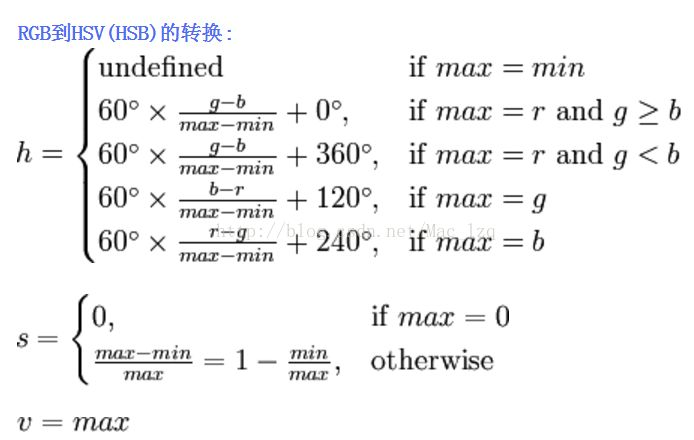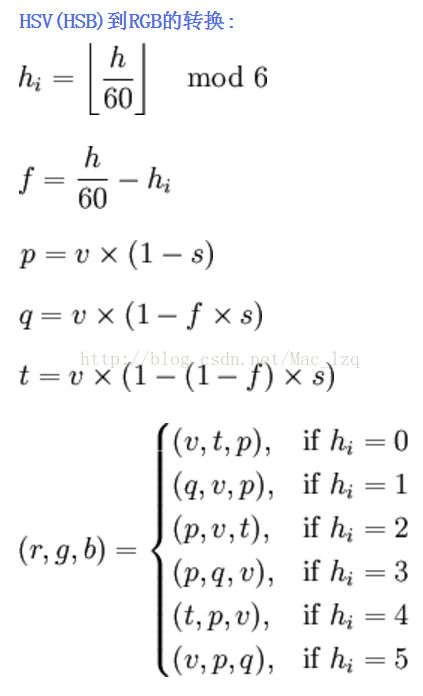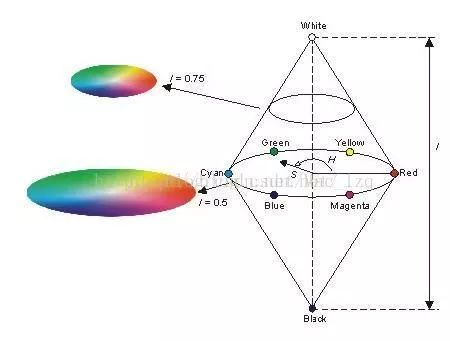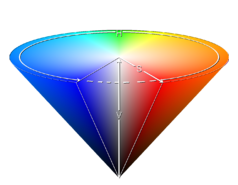(1) H和S分量与人感受彩色的方式相似，彩色图像中的每一个均匀性彩色区域都对应一个相一致的色度和饱和度，色度和饱和度能够被用来进行独立于亮度的彩色区域分割。

(2) I分量与颜色信息无关。

Lab 颜色空间

Lab颜色空间是由CIE（国际照明委员会）制定的一种色彩模式。自然界中任何一点色都可以在Lab空间中表达出来，它的色彩空间比RGB空间还要大。另 外，这种模式是以数字化方式来描述人的视觉感应， 与设备无关，所以它弥补了RGB和CMYK模式必须依赖于设备色彩特性的不足。 由于Lab的色彩空间要比RGB模式和CMYK模式的色彩空间大。这就意味着，RGB、CMYK所能描述的色彩信息，在Lab颜色空间中都能得以影身寸。

Lab颜色空间取坐标Lab，其中L亮度；a的正数代表红色，负端代表绿色；b的正数代表黄色， 负端代表兰色（a,b）有L＝116f（y）-16, a＝500[f（x／0.982）-f（y）], b＝200[f（y）-f（z／1.183 ）]；其中： f（x）＝7.787x+0.138, x〈0.008856; f（x）＝（x）1／3,x〉0.008856

CIE-lab/luv色彩空间

CIE(Commission International del’Eclairage)国际标准照明委员会于1931年建立了一系列表示可见光谱的颜色空间标准。它有三个基本量，用X、Y、Z表示，通过X、Y、Z能够表示任何一种颜色，X、Y、Z的值能够利用R、G、B线性表示出来，相对于RGB颜色空间，XYZ颜色空间几乎能包含人类能够感觉到的所有颜色，但XYZ颜色空间仍然是一种不均匀的颜色空间。因此在CIE-XYZ颜色空间的基础上又有了CIE-Lab，CIE-Luv等颜色空间。国际照明委员会制定了Lab颜色空间，人类所能感觉到的任何颜色都可以在Lab颜色空间中表示出来，其颜色空间比RGB颜色空间还大，可以直接使用欧几里德距离来衡量两种颜色的差异性。这种模式是以数字化的方式来描述人的视觉感觉，它与显示器的色移、输出设备以及其他设备无关。Lab系统是一个优秀的亮度和彩色分离器，它在图像压缩方面很有用。其中L代表亮度，a的正方向代表红色，负方向代表绿色，b的正方向代表黄色，负方向代表蓝色。Lab颜色空间由XYZ转换而得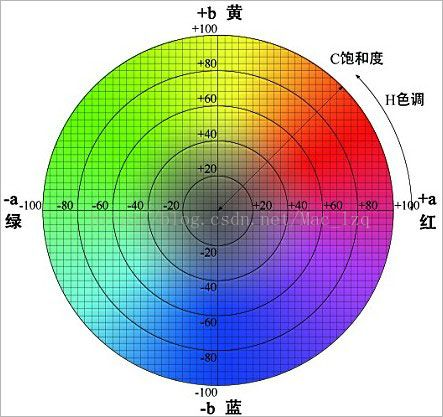YUV颜色空间

YUV、YCbCr:该颜色空间主要是基于人眼对亮度比对色度敏感这一特性而来的，将颜色分量和亮度分量分离开来。早期的黑白电视机和彩色电视机的原理也是有此而来的，具体转换公式可以参照ITU标准公式。

RGB三颜色分量转换为YUV422之后，图像的数据量便减少了1/3,如果是YUV420，则数据量便减少了一半。常用这种转换后的数据进行图像压缩编码。

CMYK颜色空间

CMYK(cyan,magenta,yellow)颜色空间应用于印刷工业,印刷业通过青©、品(M)、黄(Y)三原色油墨的不同 网点面积率的叠印来表现丰富多彩的颜色和阶调，这便是三原色的CMY颜色空间。实际印刷中，一般采用青 ©、品(M)、黄(Y)、黑(BK)四色印刷，在印刷的中间调至暗调增加黑版。当红绿蓝三原色被混合时，会产生 白色，但是当混合蓝绿色、紫红色和黄色三原色时会产生黑色。既然实际用的墨水并不会产生纯正的颜色， 黑色是包括在分开的颜色，而这模型称之为CMYK。CMYK颜色空间是和设备或者是印刷过程相关的，则工艺方法、 油墨的特性、纸张的特性等，不同的条件有不同的印刷结果。所以CMYK颜色空间称为与设备有关的表色空间。 而且，CMYK具有多值性，也就是说对同一种具有相同绝对色度的颜色，在相同的印刷过程前提下，可以用分种 CMYK数字组合来表示和印刷出来。这种特性给颜色管理带来了很多麻烦，同样也给控制带来了很多的灵活性。 在印刷过程中，必然要经过一个分色的过程，所谓分色就是将计算机中使 用的RGB颜色转换成印刷使用的CMYK 颜色。在转换过程中存在着两个复杂的问题，其一是这两个颜色空间在表现颜色的范围上不完全一样，RGB的 色域较大而CMYK则较小，因此就要进行色域压缩；其二是这两个颜色都是和具体的设备相关的，颜色本身没有 绝对性。因此就需要通过一个与设备无关的颜色空间来进行转换，即可以通过以上介绍的XYZ或LAB色空间来 进行转换。

CMY颜色空间

CMY是一种颜料混合配色体系

RGB是一种光混合配色体系C - Cyan青 〈互补色〉 R - Red 红 M - Magenta 品红 〈互补色〉 G - Green 绿 Y - Yellow 黄 〈互补色〉 B - Blue 蓝

·C和R相反，M和G相反，Y和B相反

HSL颜色空间HSL(hue,saturation,lightness)颜色空间，这个颜色空间都是用户台式机图形程序的颜色表示， 用六角形锥体表示自己的颜色模型。

HSB颜色空间HSB(hue,saturation,brightness)颜色空间，这个颜色空间都是用户台式机图形程序的颜色表示， 用六角形锥体表示自己的颜色模型。

Ycc颜色空间 柯达发明的颜色空间,由于PhotoCd在存储图像的时候要经过一种模式压缩，所以 PhotoCd采用了 Ycc颜色空间，Ycc空间将亮度作由它的主要组件，具有两个 单独的颜色通道，采用Ycc颜色空间 来保存图像，可以节约存储空间。

XYZ颜色空间国际照明委员会(CIE)在进行了大量正常人视觉测量和统计,1931年建立了"标准色度观察者"， 从而奠定了现代CIE标准色度学的定量基础。由于"标准色度观察者"用来标定光谱色时出现负刺激值，计算不便，也不易理解，因此1931年CIE在RGB 系统基础上，改用三个假想的原色X、Y、 Z建立了一个新的色度系统。将它匹配等能光谱的三刺激值，定名为"CIE1931 标准色度观察者 光谱三刺激值"，简称为"CIE1931标准色度观察者"。这一系统叫做"CIE1931标准色度系统"或称为" 2° 视场XYZ色度系统"。CIEXYZ颜色空间稍加变换就可得到Yxy色彩空间，其中Y取三刺激值中Y的值， 表示亮度，x、y反映颜色的色度特性。定义如下：在色彩管理中，选择与设备无关的颜色空间是 十分重要的，与设备无关的颜色空间由国际照明委员会(CIE)制定，包括CIEXYZ和CIELAB两个标准。 它们包含了人眼所能辨别的全部颜色。而且，CIEYxy测色制的建立给定量的确定颜色创造了条件。 但是，在这一空间中，两种不同颜色之间的距离值并不能正确地反映人们色彩感觉差别的大小， 也就是说在CIEYxy色厦图中，在 不同的位置不同方向上颜色的宽容量是不同的，这就是Yxy颜色空间 的不均匀性。这一缺陷的存在，使得在Yxy及XYZ空间不能直观地评价颜色。

https://promotion.aliyun.com/ntms/yunparter/invite.html?userCode=5wzgtzow
【全民云计算】 云主机低至4折
https://promotion.aliyun.com/ntms/act/qwbk.html?userCode=5wzgtzow
【阿里云新用户】 云通信专享8折
https://www.aliyun.com/acts/alicomcloud/new-discount?userCode=5wzgtzow
【商标注册服务】 低至680
https://tm.aliyun.com/?userCode=5wzgtzow

2013-04-10 14:51:37 mlkiller 阅读数 4206
• ###### Java经典算法讲解

在面试中，算法题目是必须的，通过算法能够看出一个程序员的编程思维，考察对复杂问题的设计与分析能力，对问题的严谨性都能够体现出来。一个算法的好坏，直接影响一个方法调用的性能，进而影响软件的整体性能。算法是学习所有编程语言的基础，在Java的学习过程中首先也会选择以算法起步，本次课程重点讲解Java开发中常用的基本算法。

29971 人正在学习 去看看 张中强

# 一含义

模糊（平滑）是一种常用的图片处理方式，它的作用可以用来降低噪声，还有其他用途

看一下opencv 里面的公式g(i,j)是目标坐标的像素值， f(i+k,j+l)是k,l这些地方的像素值， h(k,l)是 kernel,  我不知道怎么去准确翻译它的意义，它是过滤器的系数。

简单的按照我的思路去理解，就是一个权值，模糊的含义是将所有的像素按照一定的权值进行运算，得到一个比较均衡的结果。

# 三 算法

1 均值模糊
均值模糊很简单就是周边所有的影响都是1，求平均值即可2 高斯模糊# 四均值模糊的代码和效果

先放上均值模糊的代码
void boxblur(Mat input ,Mat &out, int x, int y)
{
// accept only char type matrices
CV_Assert(input.depth() != sizeof(uchar));

out.create(input.size(),input.type());

int nChannels = input.channels();
int nRows = input.rows;
int nCols = input.cols;

int size = x * y;
float kernel = 1.0/size;

int i,j;
uchar* p;
uchar* q;
uchar R,G,B;

for( i = x; i < nRows - x; ++i)
{
q = out.ptr<uchar>(i);
for ( j = y; j < nCols - y; ++j)
{
float sumR = 0;
float sumG = 0;
float sumB = 0;
for (int k =0; k<x;k++)
{
p = input.ptr<uchar>(i-x+k);
for(int l = 0; l < y;l++)
{
sumB += input.at<uchar>(i - x + k,(j + l - y)*nChannels) * kernel;//p[(l + j -y)*nChannels ] * kernel;
sumG += input.at<uchar>(i - x + k,(j + l - y)*nChannels + 1) * kernel;//p[(l + j -y)*nChannels + 1] * kernel;
sumR += input.at<uchar>(i - x + k,(j + l - y)*nChannels + 2) * kernel;//p[(l + j -y)*nChannels + 2] * kernel;
}
}
q[j*nChannels] = sumB;
q[j*nChannels+1] = sumG;
q[j*nChannels+2] = sumR;
}
}

}原始 opencv 本文# 五高斯模糊的代码和效果

void gaussblur(Mat input ,Mat &out, int x, int y)
{
float sigma = 1.5;
Mat kernel;
float pi = 3.1415926;

kernel.create(x ,y ,CV_32F);

float mx = x/2.0;
float my = y/2.0;


       //这里有问题，后面做修正。
for (int i =0; i< x;i++)
{
for (int j =0; j<y;j++)
{
kernel.at<float>(i,j) = exp(-1 * ((i - mx) * (i - mx) +(j - my) * (j-my) )/( 2 * sigma * sigma))/(2 * pi * sigma *sigma) ;
}
}


int nChannels = input.channels();
int nRows = input.rows;
int nCols = input.cols;

out.create(input.size(),input.type());
uchar* p;
uchar* q;
float* s;

for(int  i = x; i < nRows - x; ++i)
{
q = out.ptr<uchar>(i);
for (int j = y; j < nCols - y; ++j)
{
float sumR = 0;
float sumG = 0;
float sumB = 0;
for (int k =0; k<x;k++)
{
p = input.ptr<uchar>(i-x+k);
s = kernel.ptr<float>(k);
for(int l = 0; l < y;l++)
{
sumB += p[(l + j -y)*nChannels ] * s[l];//input.at<uchar>(i - x + k,(j + l - y)*nChannels) * kernel;//
sumG += p[(l + j -y)*nChannels + 1] *s[l];//input.at<uchar>(i - x + k,(j + l - y)*nChannels + 1) * kernel;//
sumR += p[(l + j -y)*nChannels + 2] * s[l];//input.at<uchar>(i - x + k,(j + l - y)*nChannels + 2) * kernel;
}
}
q[j*nChannels] = sumB;
q[j*nChannels+1] = sumG;
q[j*nChannels+2] = sumR;
}
}

}原始 opencv 本文float sum = 0;
for (int i =0; i< x;i++)
{
for (int j =0; j<y;j++)
{
sum+= kernel.at<float>(i,j) = exp(-1 * ((i - mx) * (i - mx) +(j - my) * (j-my) )/( 2 * sigma * sigma))/(2 * pi * sigma *sigma) ;
}
}
for (int i =0; i< x;i++)
{
for (int j =0; j<y;j++)
{
kernel.at<float>(i,j) = kernel.at<float>(i,j)/ sum ;
}
}

sigma = 0.3*((ksize-1)*0.5 - 1) + 0.8 .

2012-06-08 16:34:26 irr80 阅读数 301
• ###### Java经典算法讲解

在面试中，算法题目是必须的，通过算法能够看出一个程序员的编程思维，考察对复杂问题的设计与分析能力，对问题的严谨性都能够体现出来。一个算法的好坏，直接影响一个方法调用的性能，进而影响软件的整体性能。算法是学习所有编程语言的基础，在Java的学习过程中首先也会选择以算法起步，本次课程重点讲解Java开发中常用的基本算法。

29971 人正在学习 去看看 张中强

http://www.cnblogs.com/yingying0907/archive/2011/08/05/2128937.html

http://www.cnblogs.com/yingying0907/archive/2011/08/05/2128960.html